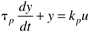### 3.5. First-Order Behavior

Many chemical processes can be modeled as first-order systems. The differential equation for a linear first-order process is often written in the following form:

Equation 3.25This can also be written aswhere the parameters (τp and kp) and variables (y and u) have the following names: τp is the process time constant (units of time), kp the process gain (units of output/input), y the output variable, and u the input variable. Taking the Laplace transform of each term (notice that we are now using lower-case variables to ...

Get Process Control: Modeling, Design, and Simulation now with the O’Reilly learning platform.

O’Reilly members experience live online training, plus books, videos, and digital content from nearly 200 publishers.